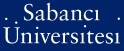# How to determine linear complexity and k-error linear complexity in some classes of linear recurring sequences

Meidl, Wilfried (2009) How to determine linear complexity and k-error linear complexity in some classes of linear recurring sequences. Cryptography and communications, 1 (1). pp. 117-133. ISSN 1936-2447 (Print) 1936-2455 (Online)

This is the latest version of this item.Preview
PDF - Requires a PDF viewer such as GSview, Xpdf or Adobe Acrobat Reader
192Kb

Official URL: http://dx.doi.org/10.1007/s12095-008-0007-6

## Abstract

Several fast algorithms for the determination of the linear complexity of d-periodic sequences over a finite field \${\mathbb F}_q\$, i.e. sequences with characteristic polynomial f(x) = x d  − 1, have been proposed in the literature. In this contribution fast algorithms for determining the linear complexity of binary sequences with characteristic polynomial f(x) = (x − 1) d for an arbitrary positive integer d, and \$f(x) = (x^2+x+1)^{2^v}\$ are presented. The result is then utilized to establish a fast algorithm for determining the k-error linear complexity of binary sequences with characteristic polynomial \$(x^2+x+1)^{2^v}\$.

Item Type: Article Linear complexity - k-error linear complexity - Algorithm - Linear recurring sequences - Stream cipher UNSPECIFIED 11479 Wilfried Meidl 29 Apr 2009 10:35 24 Feb 2010 14:24

### Available Versions of this Item

Repository Staff Only: item control page